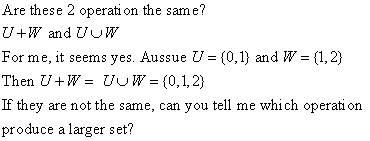# Are union and sum the same?#### Attachments

CompuChip
Homework Helper
I know what $U \cup W$ is, but how is $U + W$ defined?

Stephen Tashi
There many different meanings for mathematical notations, depending on the context. The page you gave is not talking about "+" in the context of sets. (In the context of sets, some books use $A + B$ to denote $(A \cup B) - (A \cap B)$.)

The page is talking about vector spaces. In that contex, I think $U + W$ means the vector space consisting of all vectors $h$ that can be expressed as $h = u + w$ where $u \in U$ and $w \in W$.

However, if you want to be sure of the meaning of "+" in a particular book, you must see what that book says it means. There is no "universal" meaning for it.

Deveno